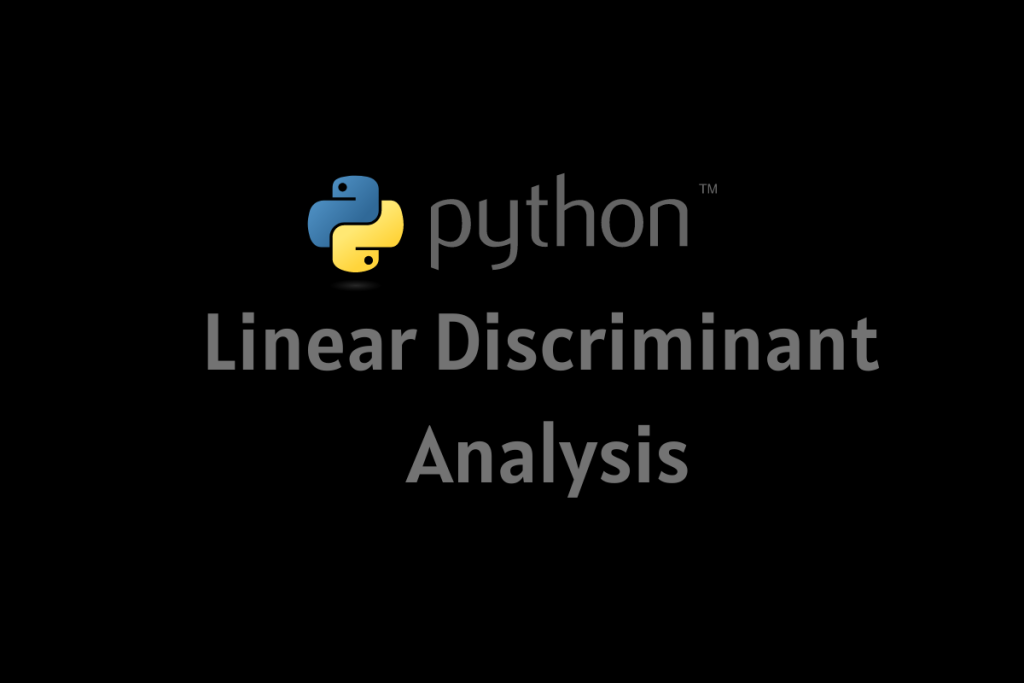# Linear Discriminant Analysis in Python – A Detailed GuideLinear Discriminant Analysis is a Dimensional Reduction Technique to solve multi-classifier problems. It is been also used for most supervised classification problems. It provides a method to find the linear combination between features of objects or classes. We can better understand this well by analyzing the steps involved in this analysis process.

Also read: Latent Dirichlet Allocation (LDA) Algorithm in Python

• Calculating Means of features of different classes.
• Calculating Within the Scatter class and Between the Scatter class.

The Scatter Class Matrix is determined by the formulaWhere c is the total no. of classes and,where Xk is the sample and n is the no. of the sample.

Between class scatter matrix is determined by; whereand• Calculating Eigen values and Eigen vectors using algorithms.
• transforming the eigenvalue and eigen vectr sinto matrix.
• once the matrix is formed, it can be used for classification ad dimensionality reduction.

Let us take we are having two classes of different features as we know the classification of these two classes using just a single feature is difficult. So we need to maximize the features to make our classification easier. That’s our goal in this topic as well.

## Applications of Linear Discriminant Analysis

Let us have a look at the applications of linear discriminant analysis.

• Classification such as classifying emails as spam, important, or anything else.
• Face recognition.
• Barcode and QR code scanning.
• Customer Identification using Artificial Intelligence in shopping platforms.
• Decision Making.
• Prediction of future prediction.

We can much better understand this by creating a model and using the same. We are taking our preloaded dataset in python which is the biochemist dataset. We will classify the marital status based on features in this dataset.

## Implementing the Linear Discriminant Analysis Algorithm in Python

To do so, from this dataset, we will fetch some data and load it into our variables as independent and dependent respectively. then we will apply the linear discriminant analysis as well to reduce the dimensionality of those variables and plot the same in the graph. Let’s follow our code snippet below.

### Step 1: Importing Modules

```import pandas as pd
from pydataset import data
from matplotlib import pyplot as plt
from sklearn.discriminant_analysis import LinearDiscriminantAnalysis
from sklearn.model_selection import train_test_split
from sklearn.metrics import classification_report
from sklearn import metrics
```

In the above code snippet, we imported our required modules as well. in case it shows any errors while importing the above modules or libraries you can install them manually in your command prompt using your pip installer.

In our code today, We will use a preloaded dataset to work on. We will fetch a dataset and load the same into a data frame df. Have a quick look at the codes below.

```#loading our preloaded dataset into a dataframe df
df = data('bioChemists')

#printing our dataframe
```

#### Step 3: Assigning values for Independent and dependent variables respectively

We will assign some value or data to our independent and dependent variables respectively. Before doing so, We will create columns of some required data and add them all into our data frame for analysis well.

```#creating columns for each value in fem and assigning 1 for positve and 0 for negative
dummy = pd.get_dummies(df['fem'])
#adding the resultant icolumns to our dataframe using concat() method
df = pd.concat([df, dummy], axis = 1)

#repeat the same for the values of mar columns
dummy = pd.get_dummies(df['mar'])
df = pd.concat([df, dummy], axis = 1)

#independent variable
x = df[['Men', 'kid5', 'phd', 'ment', 'art']]

#dependent variable
y = df['Married']
```

### Step 4: Splitting

We will use the train_test_split() method to split arrays and metrics into data subsets as trains and tests respectively (2-D array into Linear). We used the parameter `random_state=0` to get the same train and test sets after each execution.

We have passed `test_size=0.3` which means 30% of the data will be in the test set and the rest will be in the train set.

```x_train, x_test, y_train, y_test = train_test_split(x, y, test_size = 0.3, random_state = 0)
```

### Step 5: Creating Model

We will create our required linear_discriminantanalysis model and we will check its accuracy of it’s working.

```#creating our linear discrimanant analysis model
clf = LinearDiscriminantAnalysis()

#checking for the model accuracy using score method
clf.fit(x_train, y_train).score(x_train, y_train)
```

### Step 6: ROC (Receiver Operating Characteristic)

A ROC curve (Reciever Operating Characteristics) is a graph showing the performance of a classification model at all threshold levels.

This curve plots two parameters: True Positive Rate. False Positive Rate.

The function below computes the Reciever Operating Characteristics using the two reduced dimensions. Instead of plotting the linear reduced variables, We will only plot the ROC curve for the same.

```fpr, tpr, threshold = metrics.roc_curve(y_test, y_pred)

#calculating area under the curve(AUC)
auc = metrics.auc(fpr, tpr)
auc
```

### Step 7: Plotting the data using pyplot

Now We will plot the Receiver Operating Characteristic curve for the True positive rate and false positive rate obtained from the reduced dimensions for both dependent and independent variables respectively.

```#plotting our ROC curve using above terminologies
plt.title("Linear Discriminant Analysis")

plt.clf()

#plotting for roc curve
plt.plot(fpr, tpr, color="navy", linestyle="--", label = "roc_curve = %0.2f"% auc)
plt.legend(loc = "upper center")

#assigning the axis values
plt.plot([0,1.5], [0,1.5], ls = '-', color="red")

plt.xlabel("False Positive Rate")
plt.ylabel("True Positive Rate")
```

## Summary

Today We covered a sample Linear Discriminant Analysis Model. Hope you guys must have learned it with our code snippet. We must visit again with some exciting topics.